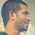# System Analysis and Design Set 3

## Questions 21 to 30

 21 Which of the following relationships are used in a use-case diagram? (a)  Communication                (b)  Uses                      (c)  Extends (d)  Both (b) and (c) above     (e)  All (a), (b) and (c) above. 22 Which of the following are UML interaction diagrams? (a)  Activity diagram                                                                            (b)  sequence diagram                                                                                               (c)  collaboration diagram (d)  class diagram                                                                                 (e)  Both (b) and (c) above. 23 The vertical dimension of a sequence diagram represents (a)  time           (b)  objects      (c)  lines           (d)  messages   (e)  methods. 24 Which of the following component is used to clarify which actor performs which activity in an activity diagram? (a)  forks          (b)  joins          (c)  swimlanes (d)  state          (e)  note. 25 Which of the following statements is not true regarding activity diagram? (a)        A solid filled circle represents the final state (b)        The final state is shown using so called bull’s eye symbol (c)        Transitions can branch and merge (diamond) – alternative computation threads (d)       Transitions can fork and re-join (bar line) –concurrent (parallel) computation threads (e)     Activity diagram without concurrent processes resembles a conventional flowchart. 26 Which of the following diagrams model the physical components of the system? (a)  use case diagram               (b)  collaboration diagram (c)  class diagram                    (d)  component diagram (e)  deployment diagram. 27 Which of the following represent the built-in extensibility mechanism of the UML? (a)  associations                       (b)  relationships                         (c)  stereotypes (d)  comments             (e)  notations. 28 Noun-Phrase Approach and CRC Approach are used to identify (a)  classes       (b)  Use cases  (c)  objects       (d)  collaborators         (e)  Actors. 29 The testing of software without the knowledge of source code is called (a)  white box testing              (b)  black box testing                   (c)  gray box testing (d)  glass box testing               (e)  blue box testing. 30 The largest percentage of total life cycle cost of software is (a)  analysis costs              (b)  design costs                (c)  maintenance costs (d)  coding costs         (e)  testing costs.

#### Answers

 21 Answer :       (e) Reason:    All the given relationships are used in the use-case diagram. 22 Answer :       (e) Reason:    sequence and collaboration diagrams are called UML interaction diagrams 23 Answer :       (a) Reason:    sequence diagram has two dimensions. The vertical denotes time and the horizontal denotes objects. 24 Answer :       (c) Reason:    swimlanes are used in activity diagrams to specify which actors are responsible for which activities. 25 Answer :       (a) Reason:    A solid circle represents the initial state. 26 Answer :       (d) Reason:    Component diagram models the physical components of the system. 27 Answer :       (c) Reason:    Stereo types represent the built-in extensibility mechanism of UML. 28 Answer :       (a) Reason:    Noun-Phrase Method and (RC Approach are used to identify classes. 29 Answer :       (b) Reason:    The testify of software without knowing the source code is called Black-box testing. 30 Answer :       (c) Reason:    maintenance costs form the largest percentage of total life cycle cost of software.

<< PrevNext >>

#### 1 comment :

1.For the question 21, can the answer be A,B,C as there can be a communication use case diagram drawn by someone ?